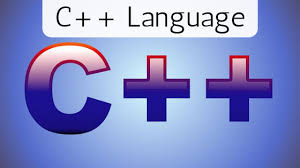### Programming in C++ (Basics)This course is an introduction to Programming using C++. This course will introduce students to basic computer concepts i.e. programs, software, input, output, processing, and a compiler. Student will learn how to write first program in C++. Basic computer programming such as Data Types, Variables, sequential programming, condition programing, decision making, looping, Array and functions